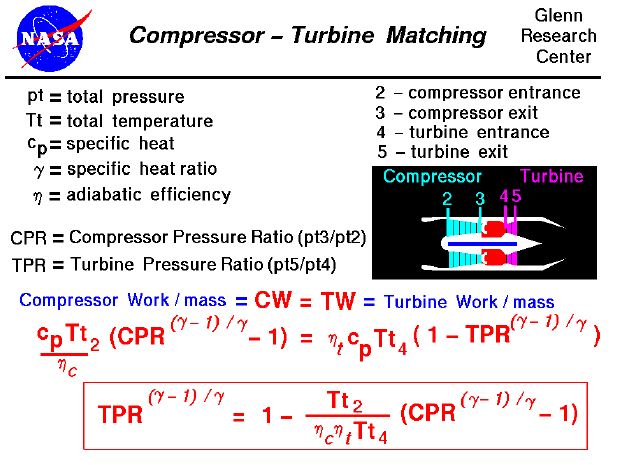Most modern passenger and military aircraft are powered by gas turbine engines, also called jet engines. The first and most simple type of gas turbine is the turbojet. In the turbojet engine, large amounts of the surrounding air are brought into the engine through the inlet. The air pressure (and temperature) are increased by the compressor, which performs work on the flow. In the burner the air is mixed with a small amount of fuel and ignited. The hot exhaust is then passed through the turbine. The turbine takes some energy out of the hot exhaust, but there is enough energy left over to provide thrust to the jet engine by increasing the velocity through the nozzle.

Separate pages describe the thermodynamics and performance aspects of the inlet, compressor, burner, turbine, and nozzle. The pressure variation EPR and temperature variation ETR through the engine can be determined if we know the individual component performances. The total engine performance ( thrust and fuel consumption) can then be easily determined. The task is simplified (a little) because the compressor and turbine are coupled together by the main shaft. The work done by the turbine must equal the work required by the compressor. On this slide we reproduce the work equations from the component slides and then simplify the equation to solve for the turbine pressure ratio (TPR) in terms of the compressor pressure ratio (CPR), the total temperature at the compressor face (Tt2) or free stream total temperature, and the total temperature at the turbine entrance (Tt4).

Compressor Work Equation: CW = cp * Tt2 * (CPR ^((gam - 1) / gam) - 1) / nc

Where cp is the specific heat coefficient at constant pressure, gam is the ratio of specific heats, and nc is the compressor efficiency.

Turbine Work Equation: TW = nt * cp * Tt4 * (1 - TPR ^ ((gam -1) / gam))

Equating and solving for TPR: TPR ^ ((gam -1) / gam) = 1 - Tt2 * (CPR ^ ((gam -1) / gam) - 1) / (nc * nt * Tt4)

We can use this equation in two ways. We can specify the pressure increase across the compressor to determine the pressure loss through the turbine. Or, if we know the pressure loss across the turbine, we can solve for the pressure increase through the compressor. The first use of the equation is employed in preliminary engine design, while the second use is employed in determining "off-design" performance.

Every engine is designed around some set of desired conditions. Those conditions might include the size of the engine, the thrust at takeoff, or the maximum thrust at some prescribed altitude. At the design condition, we can select values of the important thermodynamic variables: the maximum burner temperature, the CPR, and the nozzle area. All other flight conditions (speed and altitude) and throttle settings will be "off-design." For an existing engine, the pressure loss across the turbine can be determined by the airflow through the nozzle and the throttle setting (Tt4), as shown on a separate slide. From the above equation, we can then determine the compressor pressure ratio.Guided Tours